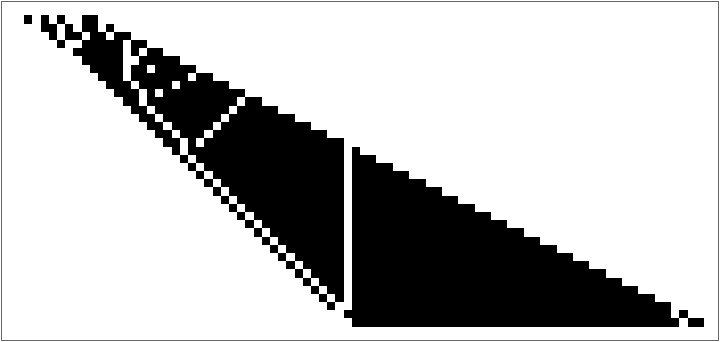#Function Repository Resource:

# CatalanUnrank

Find the totally balanced binary sequence for a given rank

Contributed by: Ed Pegg Jr
 ResourceFunction["CatalanUnrank"][n,rank] gives the totally balanced binary sequence with n ones and the given rank.

## Details and Options

A binary sequence is considered totally balanced if the number of zeros is at least as large as the number of ones as one progresses from left to right in a list of zeros and ones, and the total counts are equal (implying the first element must be zero and the last element one).
For n ones there are Cn totally balanced binary sequences, where Cn is the Catalan number.
The value returned is the member at position rank in the set of all possible balanced sequences with n zeros and n ones, ordered according to a certain enumeration scheme.
Given a balanced sequence of zeros and ones, its position in the enumeration of all such can be found using the resource function CatalanRank.
Brackets in a computer program must be balanced. One can think of a proper bracketing as having left brackets corresponding to zeros and right brackets to ones in a balanced binary sequence.

## Examples

### Basic Examples (2)

Here is the number of totally balanced binary sequences with five 1's:

 In:=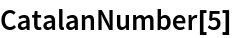Out=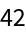Use CatalanUnrank to find the 20th totally balanced binary sequence with five 1's:

 In:=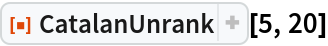Out=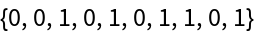Show all totally balanced binary sequences with five 1’s:

 In:=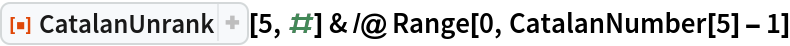Out=### Neat Examples (1)

The first few balanced binary sequences of rank 40:

 In:=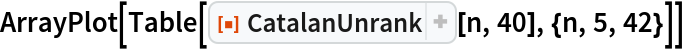Out=